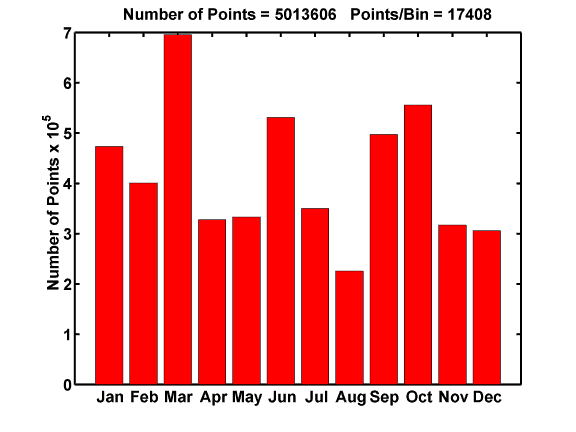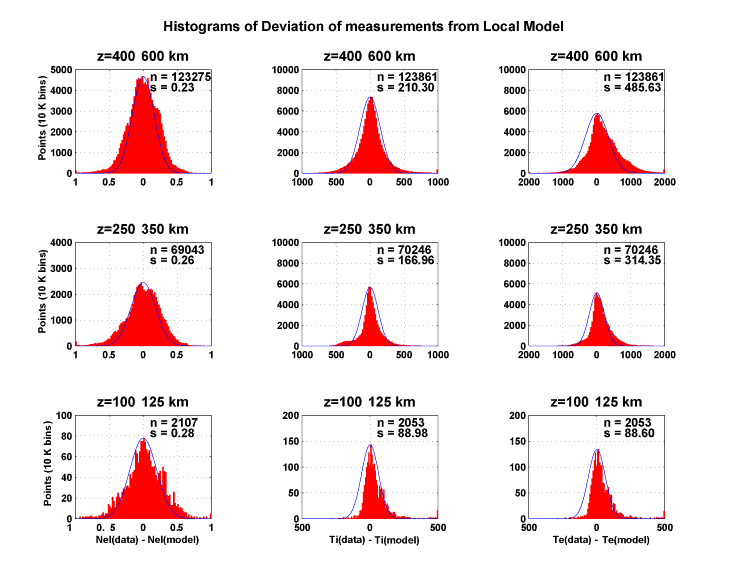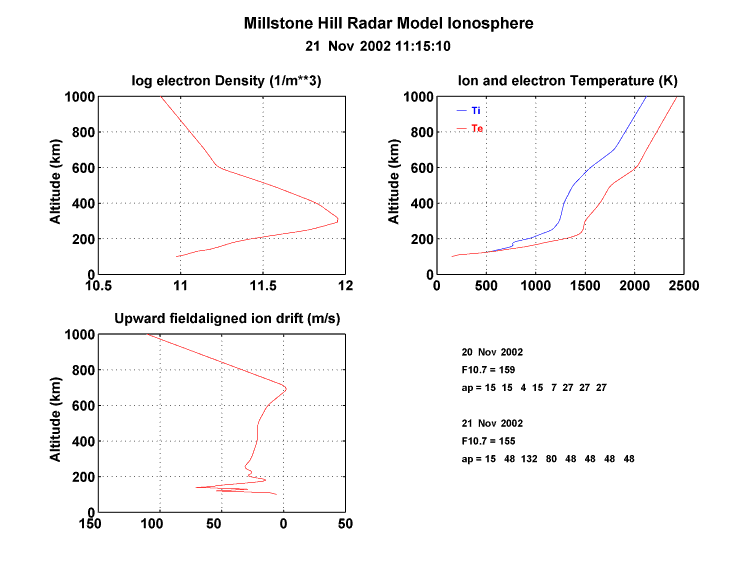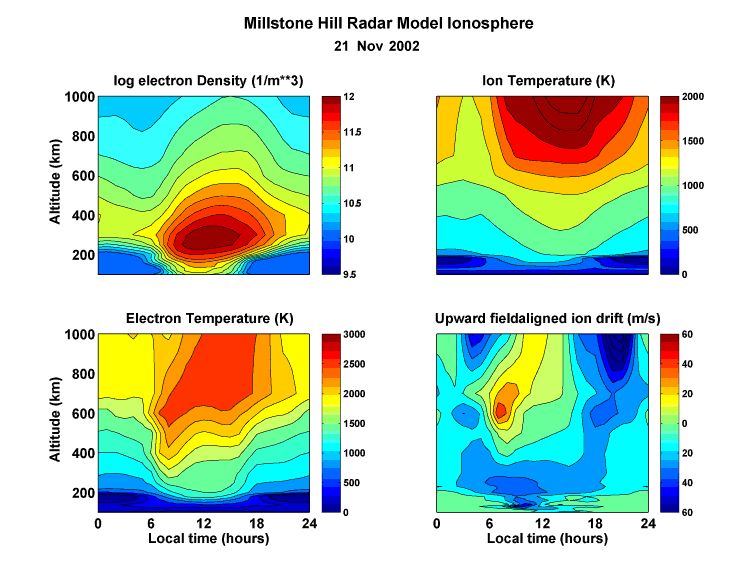# Local Empirical Model of the E and F Region Ionosphere Based on 30 Years of Millstone Hill Incoherent Scatter Data

## Introduction

Improved specifications and predictions of the ionosphere/thermosphere system are an important objective of the National Space Weather Program. As a contribution toward meeting this objective, we are developing a series of empirical models of the average behavior and variability of key parameters which characterize the ionosphere/thermosphere system. Among these is a local model of the E and F-regions above Millstone Hill. This model combines and extends previous models of F-region density and temperature and F-region ion drifts.

## Data

The model is based on Millstone Hill data collected between 1970 and 2001, and archived in the Madrigal Database (http://www.openmadrigal.org). The measured parameters are electron density (Ne), ion temperature (Ti), electron temperature (Te) and line-of-sight ion drift (Vi). Each measurement was passed through a quality-control filter and the data which passed this filter were written to twelve ASCII flat files, one for each month. Altogether there were 5,013,606 measurements, most of which included all four parameters.## Data Distribution

The large volume of data used in each fit profile permits us to investigate the distribution of the data about the fit. These can then be used to validate the least-squares fitting and to provide an estimate of the model accuracy. The figure shows example distributions for log10(Ne), Ti and Te in three different altitude ranges. In addition to the distribution histogram, each figure contains the number of points in the distribution (n) and the standard deviation (\sigma). The solid line is a Gaussian with the same standard deviation as the distribution. Least squares yields the maximum likelihood estimate if the data are Gaussian distributed, and this is seen to be a fairly good assumption. In most cases the model estimate is quite close to the histogram maximum likelihood value, even when the distribution deviates noticeably from a Gaussian. Most distributions have larger wings than a Gaussian, and Te is typically skewed toward larger values, particularly at high attitudes The latter is a well-known consequence of the non-linear and highly-correlated dependence of the incoherent scatter spectrum on Ti and Te.## Model Formulation

The data were binned by month and local time in 1-hour increments. Least squares fits for model parameter profiles were then computed in each of the 288 bins. The model parameters are Nel (log10 electron density), Ti, Te, Vi7 (geomagnetic-field-aligned upward ion velocity), Vi8 (apex southward perpendicular velocity) and Vi9 (apex eastward perpendicular velocity), where Nel etc are the CEDAR parameter mnemonic for each parameter. The fitted profiles are piecewise-linear with nodes at 100, 110, 120, 130, 140, 160, 180, 200, 225, 250, 300, 350, 400, 500, 600, 700 and 1000 km. The perpendicular velocity components are assumed to be E×B drifts, and were held constant above 130 km. The parameters are assumed to be linear in F10.7 for the previous day and the Ap index for the previous 3 hour period. The least squares fit yields a total of 4896 fit coefficients for Nel, Ti, Te and vi7, and 1728 fit coefficients for Vi8 and Vi9. A 3×3 median filter in local time and season was then applied to these coefficients. Finally, since Millstone Hill cannot make measurements in the nighttime E-region, the daytime E-region data are fit with a diurnal harmonic yielding interpolated model coefficients for the nighttime E-region.

Millstone Hill local model plots for the current date, time and geophysical conditions are available at http://madrigal.haystack.edu/models/vmhr/. Regional model plots are also available here. These plots are updated every 15 minutes. The models are evaluated using recent measured and predicted values of F10.7 and ap (or Ap for the local time vs altitude plot). When a measured value of ap is not available, the last measured value is used.